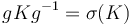# Automorph-conjugacy is transitive

This article gives the statement, and possibly proof, of a subgroup property (i.e., automorph-conjugate subgroup) satisfying a subgroup metaproperty (i.e., transitive subgroup property)
View all subgroup metaproperty satisfactions | View all subgroup metaproperty dissatisfactions |Get help on looking up metaproperty (dis)satisfactions for subgroup properties
Get more facts about automorph-conjugate subgroup |Get facts that use property satisfaction of automorph-conjugate subgroup | Get facts that use property satisfaction of automorph-conjugate subgroup|Get more facts about transitive subgroup property

## Statement

Suppose$H \le K \le G$ are groups such that$H$ is an automorph-conjugate subgroup of$K$, and$K$ is an automorph-conjugate subgroup of$G$. Then,$H$ is an automorph-conjugate subgroup of$G$.

## Proof

This proof uses a tabular format for presentation. Provide feedback on tabular proof formats in a survey (opens in new window/tab) | Learn more about tabular proof formats|View all pages on facts with proofs in tabular format

Given: Groups$H \le K \le G$ such that$H$ is an automorph-conjugate subgroup of$K$ and$K$ is an automorph-conjugate subgroup of$G$. An automorphism$\sigma$ of$G$.

To prove: There exists$x \in G$ such that$\sigma(H) = xHx^{-1}$

Proof:

Step no. Assertion/construction Given data used Previous steps used Explanation
1$\sigma(H) \le \sigma(K)$$H \le K$ given-direct
2 There exists$g \in G$ such that$g K g^{-1} = \sigma(K)$$K$ is an automorph-conjugate subgroup of$G$$\sigma$ is an automorphism of$G$
given-direct
3 Denote by$c_g$ the map$u \mapsto gug^{-1}$. Then$c_g^{-1} \circ sigma$ is an automorphism of$G$ that restricts to an automorphism of$K$. Step (2) direct from the step
4 There exists$k \in K$ such that$(c_g^{-1} \circ \sigma)(H) = kHk^{-1}$.$H$ is an automorph-conjugate subgroup of$K$ Step (3) Step-given combination direct
5 Setting$x = gk$, we get that$\sigma(H) = xHx^{-1}$ Step (4) Simple algebraic manipulation gives$\sigma(H) = c_g(kHk^{-1})$$= gkHk^{-1}g^{-1} = (gk)H(gk)^{-1}$